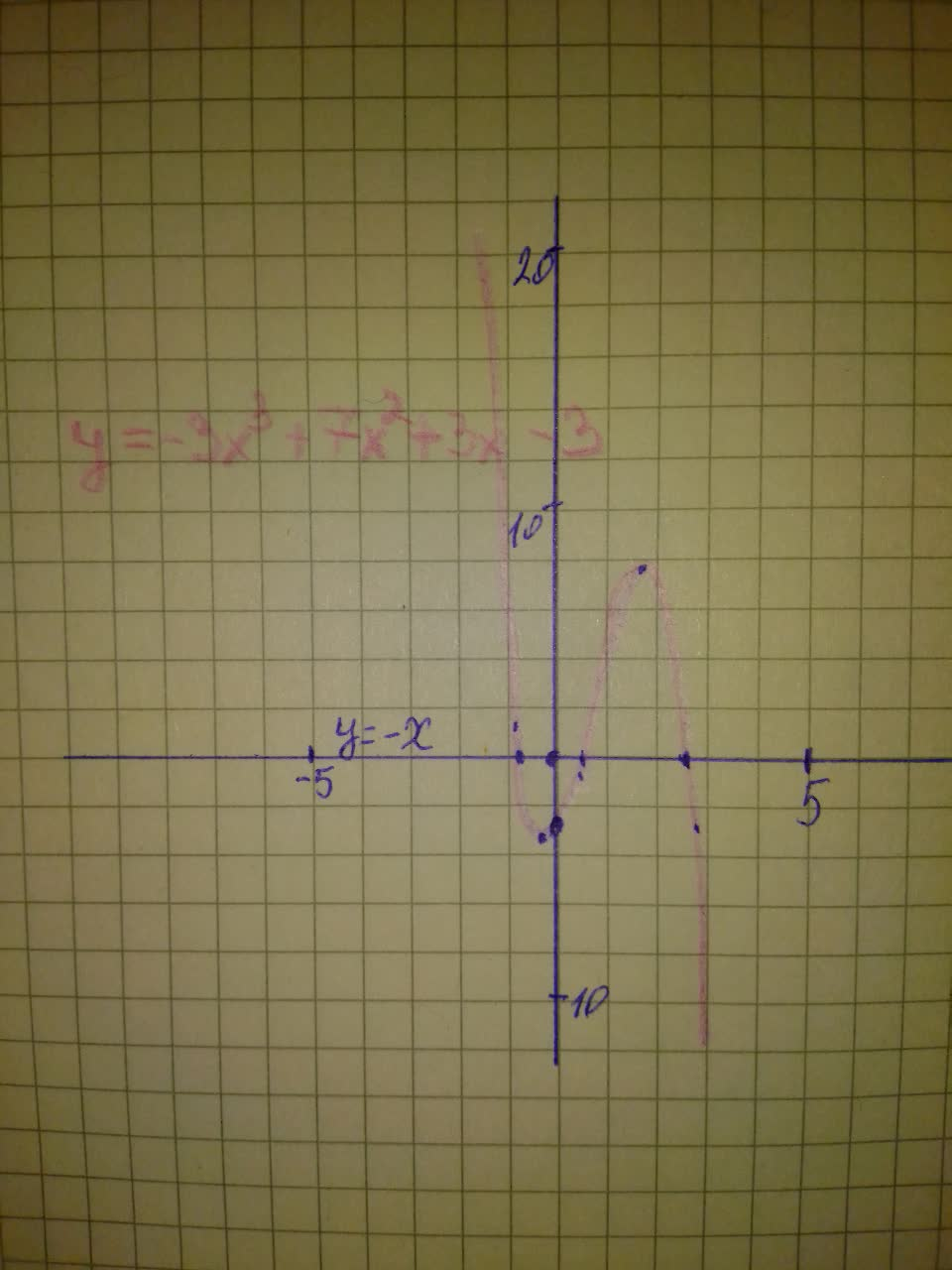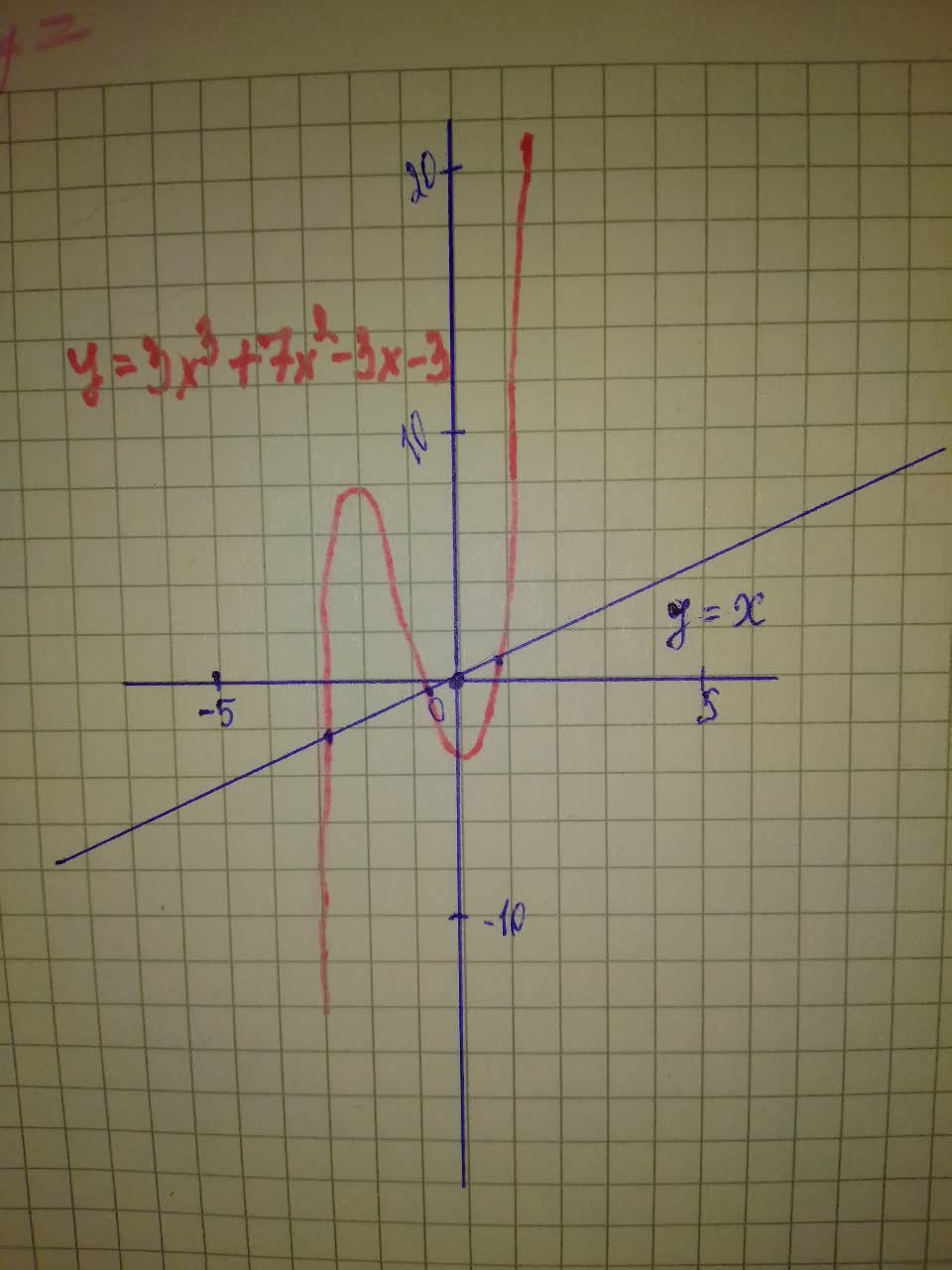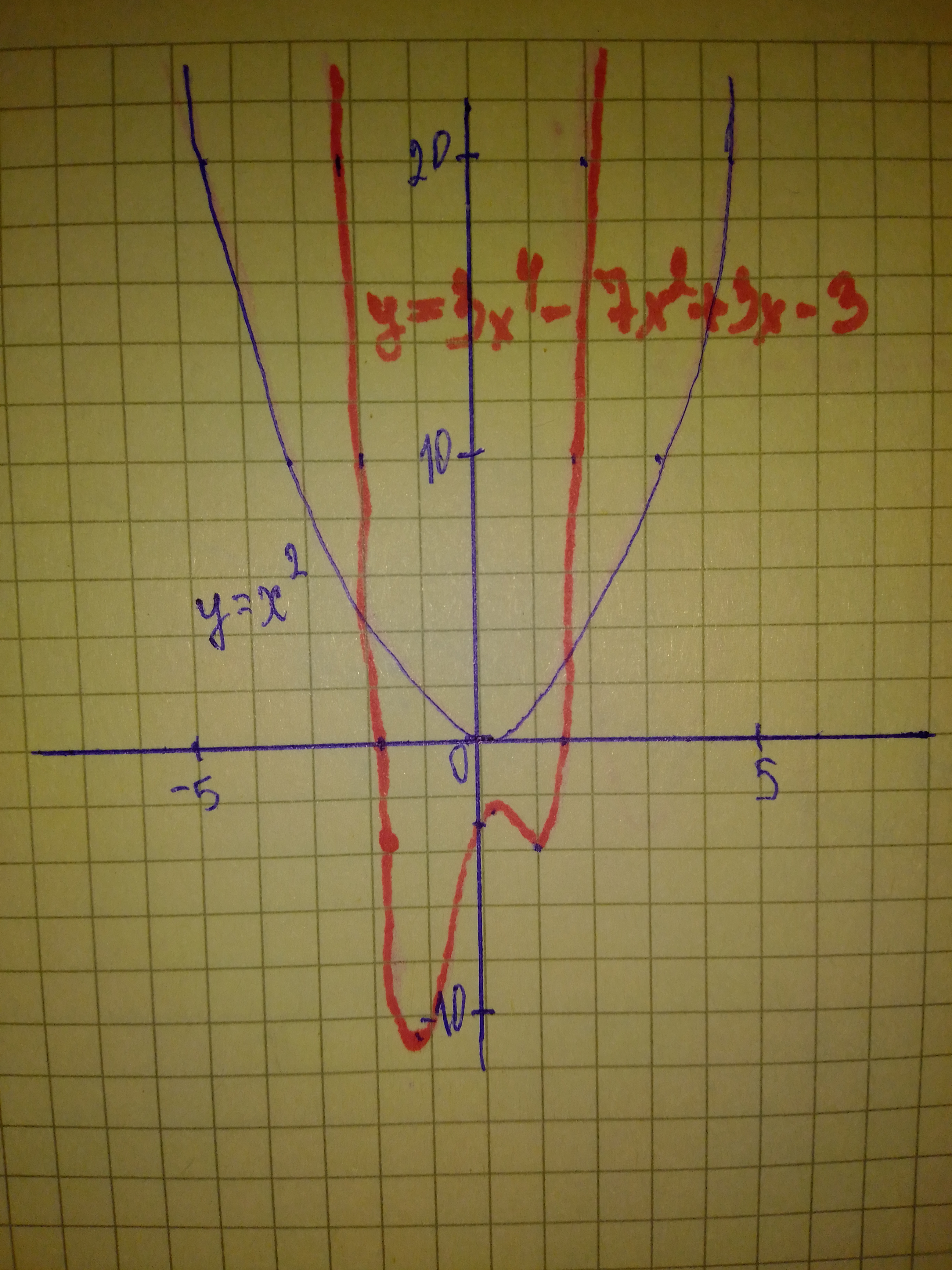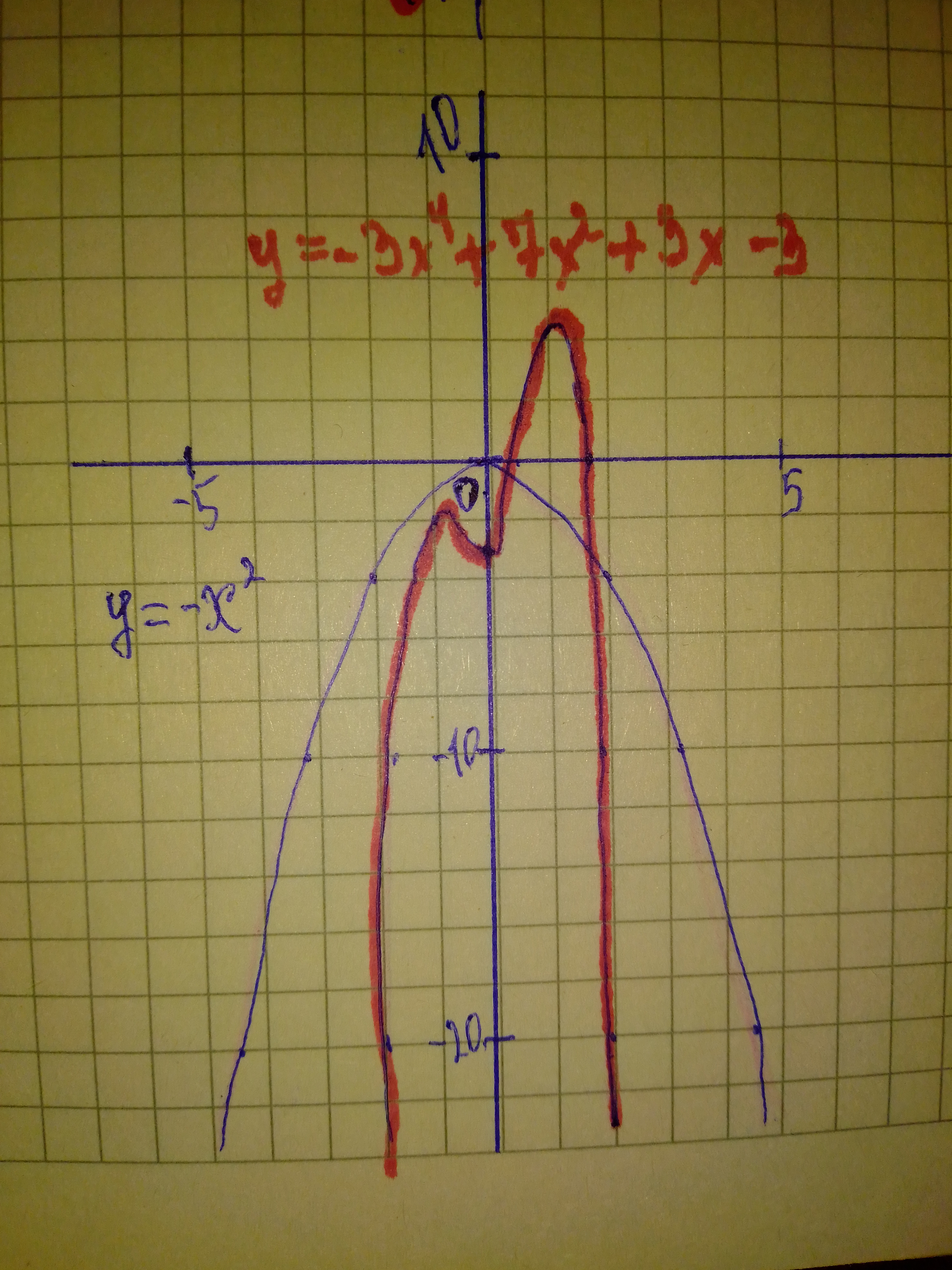# Describe the similarities between a) the lines y = x and y = -x and the graphs of other odd-degree polynomial functions b) the parabolas y=x^{2} and y=-x^{2} and the graphs of other even-degree polynomial functionsarenceabigns 2021-01-07 Answered
Describe the similarities between a) the lines $y=x\phantom{\rule{1em}{0ex}}\text{and}\phantom{\rule{1em}{0ex}}y=-x$ and the graphs of other odd-degree polynomial functions b) the parabolas $y={x}^{2}\phantom{\rule{1em}{0ex}}\text{and}\phantom{\rule{1em}{0ex}}y=-{x}^{2}$ and the graphs of other even-degree polynomial functions
You can still ask an expert for help

• Questions are typically answered in as fast as 30 minutes

Solve your problem for the price of one coffee

• Math expert for every subject
• Pay only if we can solve itBertha Stark

Step 1
The graphs of the Odd Degree Polynomial Functions will depend mainly on the Leading Coefficient.
If it is Positive the graph will expand feom quadrant 3 to quadrant 1 i.e. like to the graph of $y=x$
On the other hand, If it is Negative the graph will expand from quadrant 2 to quadrant 4 i.e. like to the graph of $y=-x$
Step 2Step 3Step 4 The Graphs of the Even Degree Polynomial Functions will depend mainly on the Leading Coefficient.
If it is Positive the graph will expand from quadrant 2 to quadrant 1 i.e. like to the graph of $y={x}^{2}$.
On the other hand, If it is Negative the graph will expand from quadrant 3 to quadrant 4 i.e. like to the graph of $y=-{x}^{2}$
Step 5Step 6a) Graph of odd-degree polynomial functions and the lines $y=x\phantom{\rule{1em}{0ex}}\text{and}\phantom{\rule{1em}{0ex}}y=-x$
b) Graph of even-degree polynomial functions and the lines $y={x}^{2}\phantom{\rule{1em}{0ex}}\text{and}\phantom{\rule{1em}{0ex}}y=-{x}^{2}$